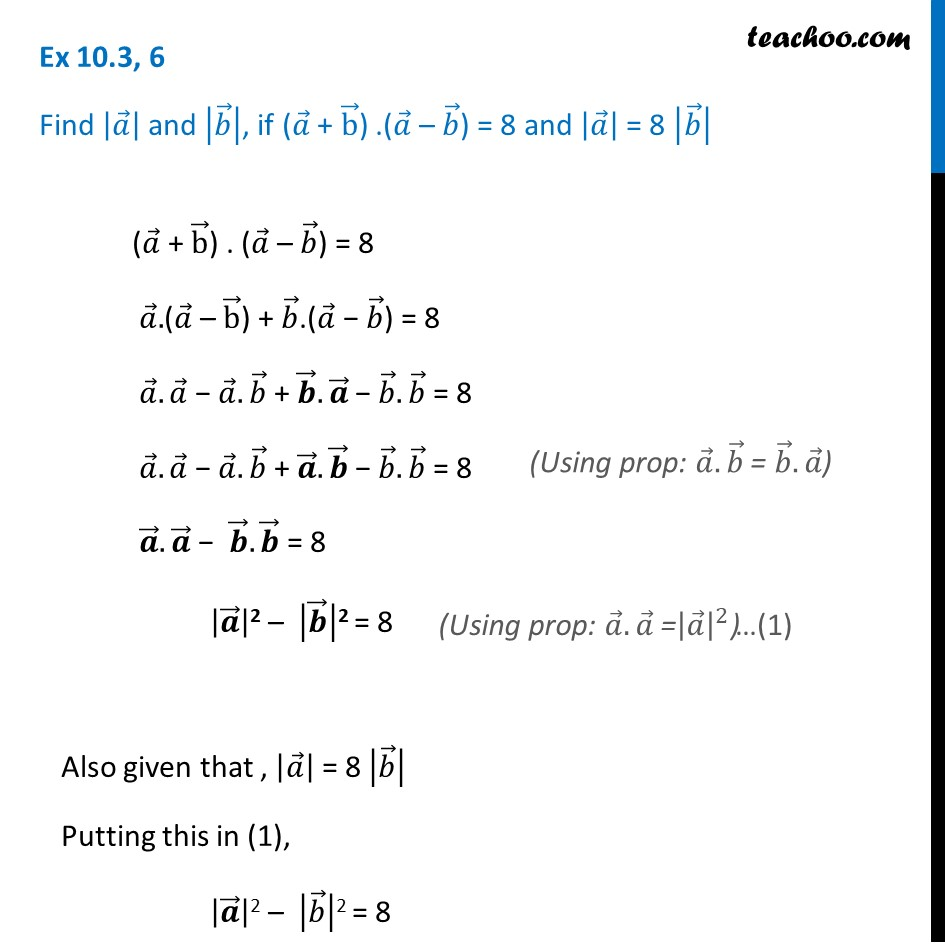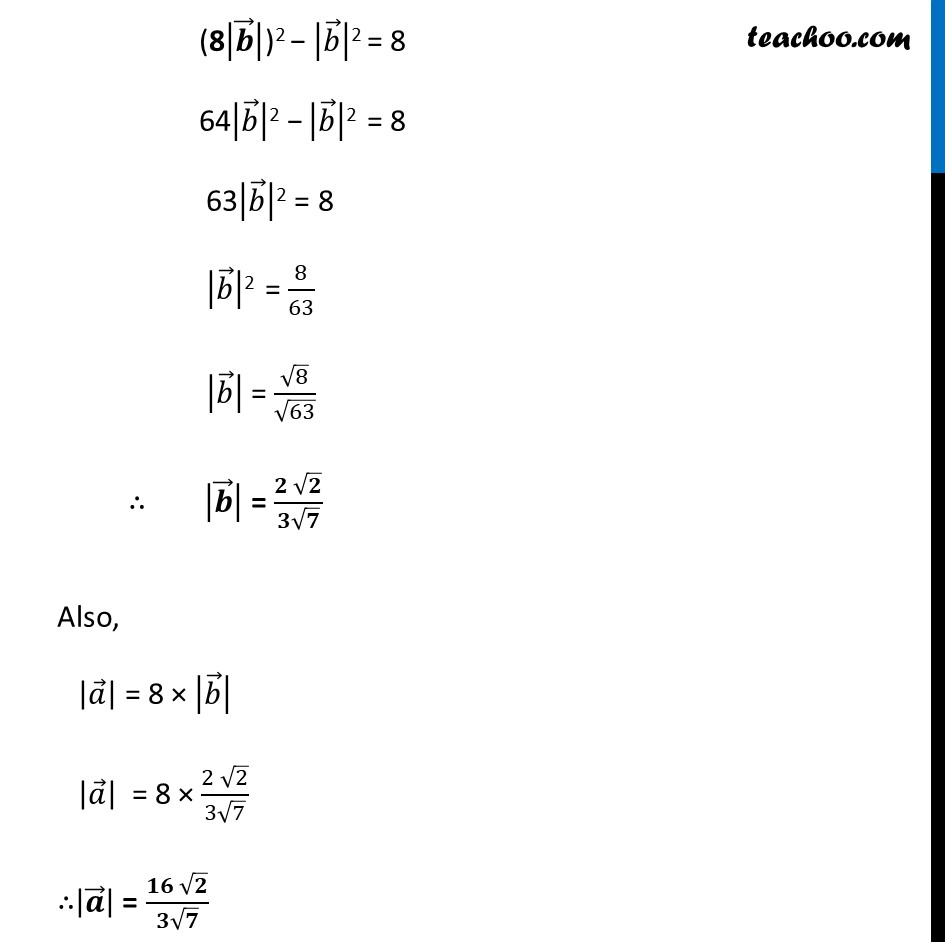Scalar product - Solving

Chapter 10 Class 12 Vector Algebra
Concept wiseLearn in your speed, with individual attention - Teachoo Maths 1-on-1 Class

### Transcript

Ex 10.3, 6 Find |𝑎 ⃗ | and |𝑏 ⃗ |, if (𝑎 ⃗ + b ⃗) .(𝑎 ⃗ – 𝑏 ⃗) = 8 and |𝑎 ⃗ | = 8 |𝑏 ⃗ | (𝑎 ⃗ + b ⃗) . (𝑎 ⃗ – 𝑏 ⃗) = 8 𝑎 ⃗.(𝑎 ⃗ – b ⃗) + 𝑏 ⃗.(𝑎 ⃗ − 𝑏 ⃗) = 8 𝑎 ⃗.𝑎 ⃗ − 𝑎 ⃗.𝑏 ⃗ + 𝒃 ⃗.𝒂 ⃗ − 𝑏 ⃗.𝑏 ⃗ = 8 𝑎 ⃗.𝑎 ⃗ − 𝑎 ⃗.𝑏 ⃗ + 𝒂 ⃗.𝒃 ⃗ − 𝑏 ⃗.𝑏 ⃗ = 8 𝒂 ⃗.𝒂 ⃗ − 𝒃 ⃗.𝒃 ⃗ = 8 |𝒂 ⃗ |2 – |𝒃 ⃗ |2 = 8 Also given that , |𝑎 ⃗ | = 8 |𝑏 ⃗ | Putting this in (1), |𝒂 ⃗ |2 – |𝑏 ⃗ |2 = 8 (Using prop: 𝑎 ⃗.𝑏 ⃗ = 𝑏 ⃗.𝑎 ⃗) (8|𝒃 ⃗ |)2 − |𝑏 ⃗ |2 = 8 64|𝑏 ⃗ |2 − |𝑏 ⃗ |2 = 8 63|𝑏 ⃗ |2 = 8 |𝑏 ⃗ |2 = 8/63 |𝑏 ⃗ | = √8/√63 ∴ |𝒃 ⃗ | = (𝟐 √𝟐)/(𝟑√𝟕) Also, |𝑎 ⃗ | = 8 × |𝑏 ⃗ | |𝑎 ⃗ | = 8 × (2 √2)/(3√7) ∴|𝒂 ⃗ | = (𝟏𝟔 √𝟐)/(𝟑√𝟕)